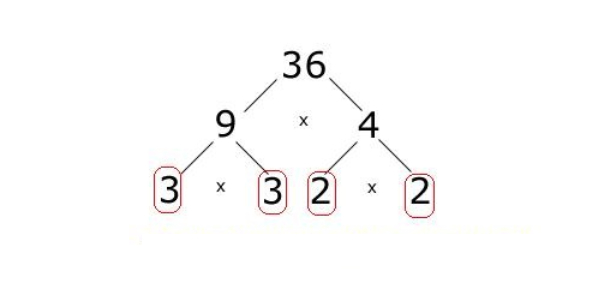# Quiz: Take The Prime Factorization Math Test!

10 Questions | Attempts: 12110
ShareSettingsTake the prime factorization math test! In the study of mathematics, the prime factorization of a number is achieved when we figure out what prime numbers multiply for us to find it. That can be as many as two numbers in an equation or as many as it possibly takes to reach it. See how well you understand this notion by taking the quiz below!

• 1.
Is 16 prime or composite?
• 2.
2    x    72    =
• 3.
23  x  3  =
• 4.
What is the prime factorization of 93?
• A.

3 x 30

• B.

3 x 31

• C.

3 x 3 x 3 x 3

• 5.
What is the prime factorization of  56?
• A.

7 x 8

• B.

2 x 4 x 7

• C.

2 x 2 x 2 x 7

• 6.
What is the prime factorization of  45?
• A.

3 x 3 x 5

• B.

5 x 9

• C.

2 x 3 x 5

• 7.
What is the prime factorization of  120?
• A.

20 x 5

• B.

2 x 2 x 3 x 5

• C.

2 x 2 x 2 x 3 x 5

• 8.
Making a factor tree is a way to demonstrate the prime factorization of a number.
• A.

True

• B.

False

• 9.
75  =  7 x 5
• A.

True

• B.

False

• 10.
9 4 = 9 x 9 x 9 x 9
• A.

True

• B.

False

## Related TopicsBack to top
×

Wait!
Here's an interesting quiz for you.